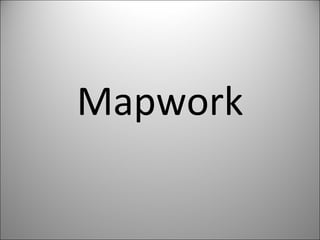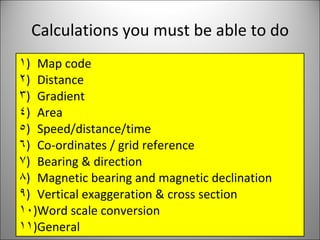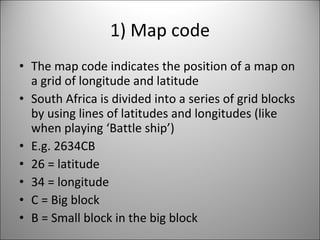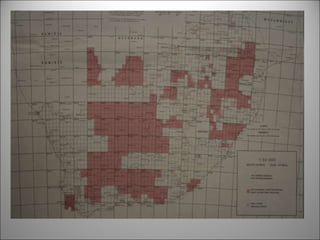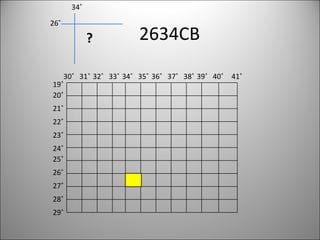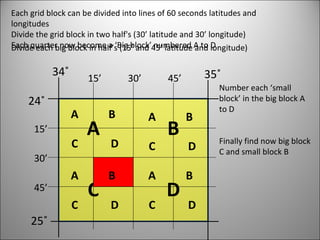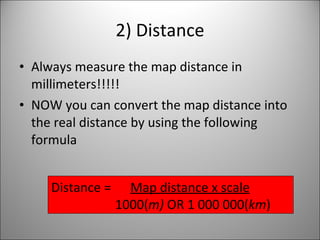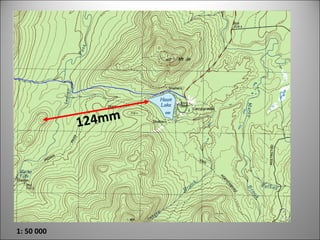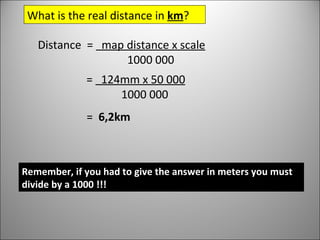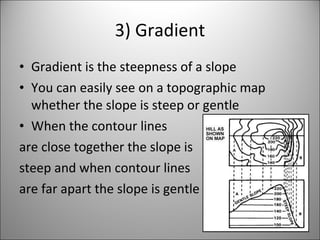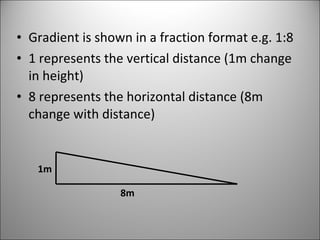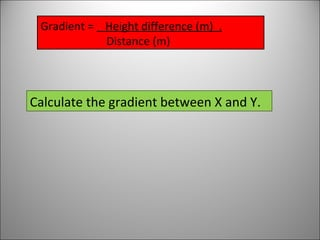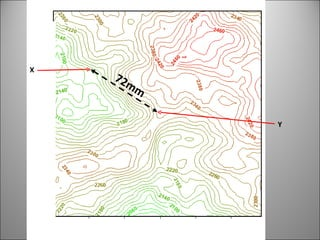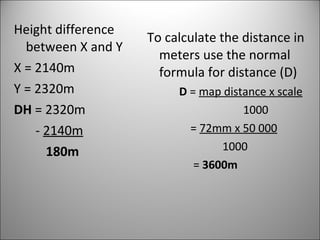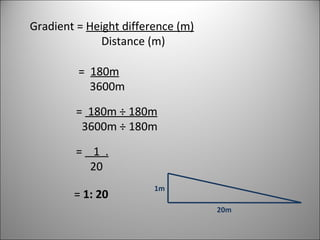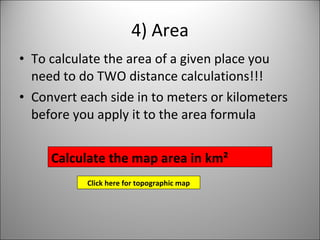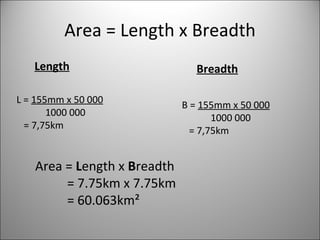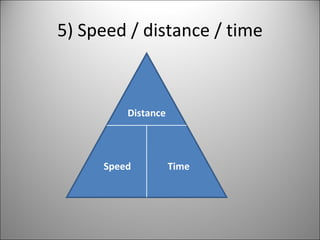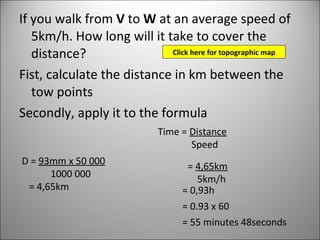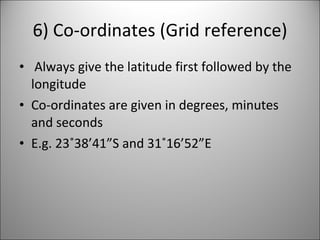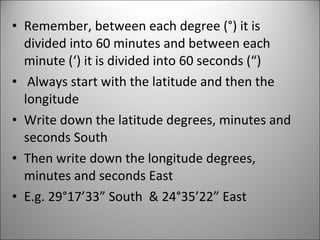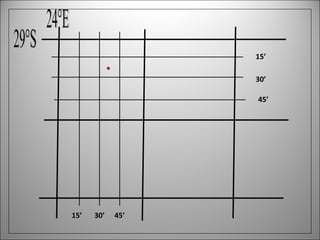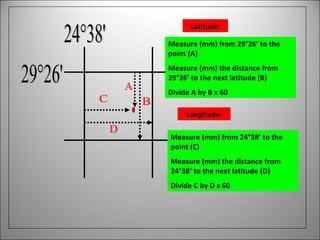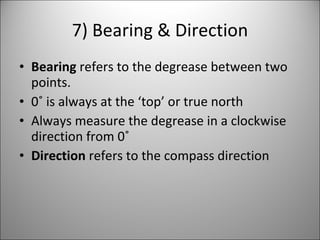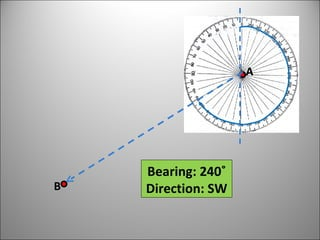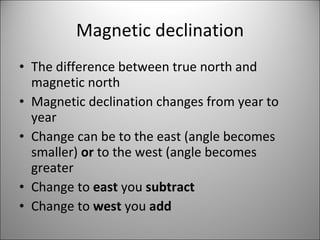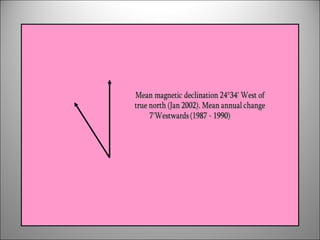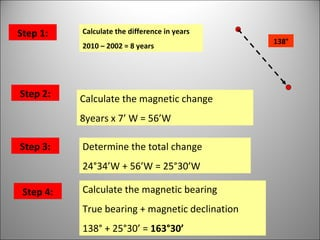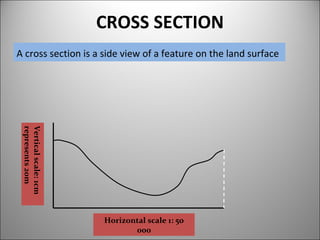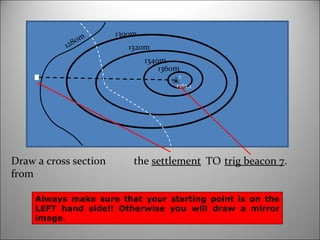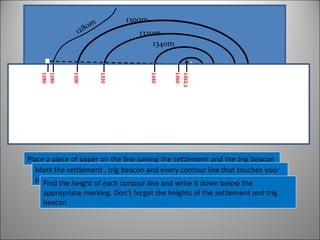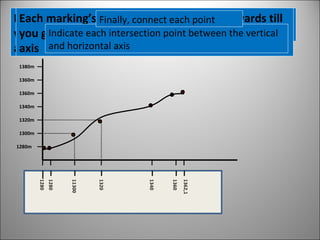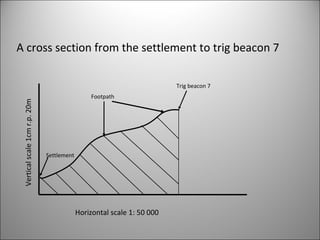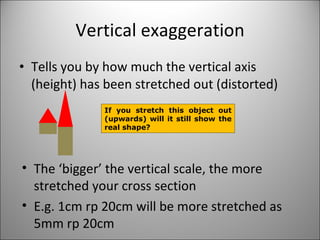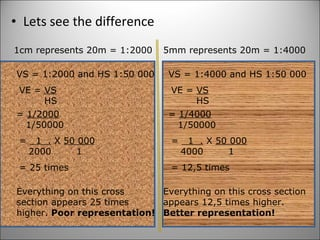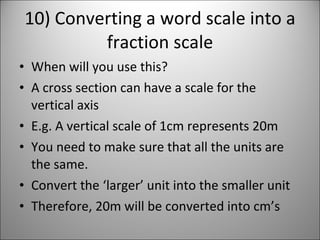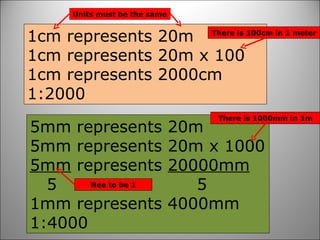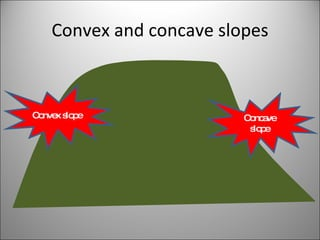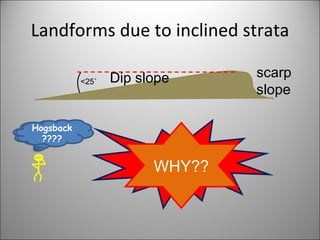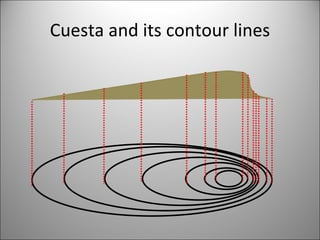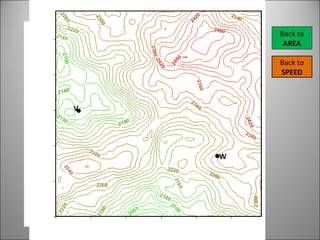1 of 42

### Mapwork calculations

1. Mapwork
2.
3. 26˚ 34˚ ? 2634CB 19˚ 20˚ 21˚ 22˚ 23˚ 24˚ 25˚ 26˚ 27˚ 28˚ 29˚ 30˚ 31˚ 32˚ 33˚ 34˚ 35˚ 36˚ 37˚ 38˚ 39˚ 40˚ 41˚
4. Each grid block can be divided into lines of 60 seconds latitudes and longitudes Divide the grid block in two half's (30’ latitude and 30’ longitude) Each quarter now become a ‘Big block’ numbered A to D 24˚ 25˚ 34˚ 35˚ Divide each big block in half’s (15’ and 45’ latitude and longitude) Number each ‘small block’ in the big block A to D Finally find now big block C and small block B 30’ 30’ 15’ 15’ 45’ 45’ A B C D A B C D A B C D A B C D A B C D
5. 124mm 1: 50 000
6. Distance = map distance x scale 1000 000 What is the real distance in km ? = 124mm x 50 000 1000 000 = 6,2km Remember, if you had to give the answer in meters you must divide by a 1000 !!!
7. Gradient = Height difference (m) . Distance (m) Calculate the gradient between X and Y.
8. 72mm X Y
9. Gradient = Height difference (m) Distance (m) = 180m 3600m = 180m ÷ 180m 3600m ÷ 180m = 1 . 20 = 1: 20 1m 20m
10. Area = Length x Breadth Length Breadth L = 155mm x 50 000 1000 000 = 7,75km B = 155mm x 50 000 1000 000 = 7,75km Area = L ength x B readth = 7.75km x 7.75km = 60.063km²
11. 5) Speed / distance / time Distance Speed Time
12. 29°S 24°E 30’ 45’ 15’ 30’ 45’ 15’
13. 29°26' 24°38' Latitude: Measure (mm) from 29°26’ to the point (A) Measure (mm) the distance from 29°26’ to the next latitude (B) Divide A by B x 60 Longitude: Measure (mm) from 24°38’ to the point (C) Measure (mm) the distance from 24°38’ to the next latitude (D) Divide C by D x 60 C D B A
14. A B Bearing: 240˚ Direction: SW
15.
16. 138° Step 1: Calculate the difference in years 2010 – 2002 = 8 years Step 2: Calculate the magnetic change 8years x 7’ W = 56’W Step 3: Determine the total change 24°34’W + 56’W = 25°30’W Step 4: Calculate the magnetic bearing True bearing + magnetic declination 138° + 25°30’ = 163°30’
17. trig beacon 7 . Draw a cross section from the settlement TO 1362,1 1340m 1320m 1300m 1280m 7 1360m Always make sure that your starting point is on the LEFT hand side!! Otherwise you will draw a mirror image. 1360m
18. 1280 1362,1 1360 1340 1320 1300 1280 Place a piece of paper on the line joining the settlement and the trig beacon Mark the settlement , trig beacon and every contour line that touches your paper Find the height of each contour line and write it down below the appropriate marking. Don’t forget the heights of the settlement and trig beacon 1362,1 1340m 1320m 1300m 1280m 7
19. Now you are going to draw the cross section Draw the horizontal axis (slightly longer than the line between the settlement and the trig beacon) Transfer the markings from the piece of paper onto the horizontal axis On the vertical axis make a mark every 20cm’s Indicate the heights on the vertical axis, starting with the lowest altitude (1280m) from horizontal axis next to the first marking Each marking’s height increase by 20m upwards till you get to the highest altitude from the horizontal axis 1280m 1300m 1320m 1340m 1360m 1360m 1380m Indicate each intersection point between the vertical and horizontal axis Finally, connect each point
20. 1362,1 1340m 1320m 1300m 1280m 7
21. A cross section from the settlement to trig beacon 7 Horizontal scale 1: 50 000 Vertical scale 1cm r.p. 20m Footpath Trig beacon 7 Settlement
22. 1cm represents 20m 1cm represents 20m x 100 1cm represents 2000cm 1:2000 5mm represents 20m 5mm represents 20m x 1000 5mm represents 20000mm 5 5 1mm represents 4000mm 1:4000 Units must be the same Nee to be 1 There is 1000mm in 1m There is 100cm in 1 meter
23. Convex and concave slopes Convex slope Concave slope
24. Landforms due to inclined strata Cuesta???? Homoclinal ridge???? Hogsback???? CUESTA WHY?? Dip slope scarp slope <25˚
25. Cuesta and its contour lines
26. Back to AREA V W Back to SPEED